Geometry

# Volume - Pyramid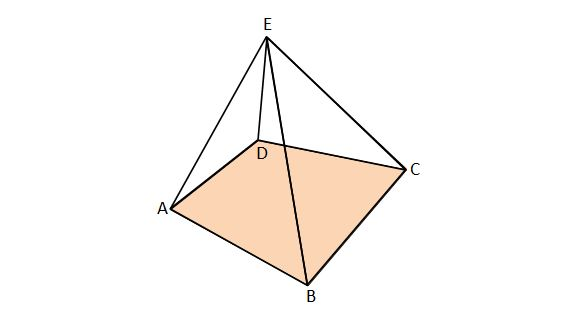Suppose the pyramid in the above diagram has a square base with side length $8\text{ cm}.$ If the volume of the pyramid is $320\text{ cm}^3,$ what is its height in $\text{cm}?$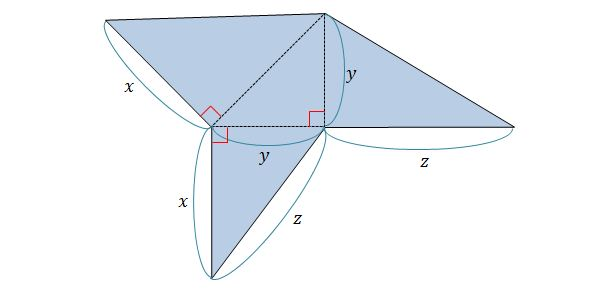If $x=6,$ $y=10$ and $z=\sqrt{136},$ what is the volume of the rigid body which can be formed by the above planar figure?

Note: The above diagram is not drawn to scale.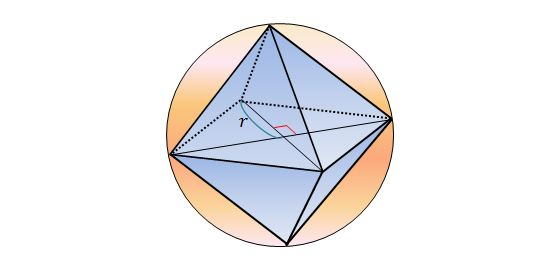If a regular octahedron is inscribed in a sphere with radius $r=8,$ as shown above, what is the volume of this regular octahedron?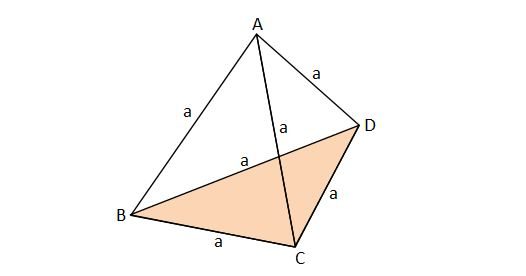What is the volume of the above tetrahedron with six identical edges of length $5 ?$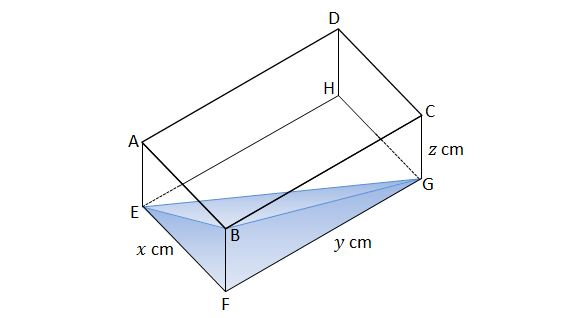If $x=6,$ $y=16,$ and $z=11,$ what is the volume of the water contained in the box, given that the water just touches the corner at $B$. Give your answer in $\text{cm}^3 ?$

The above diagram is not drawn to scale.

×